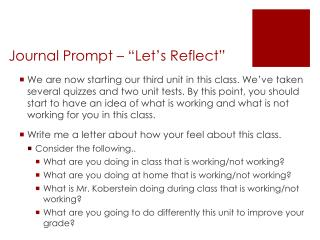# Journal Prompt – “Let’s Reflect” - PowerPoint PPT PresentationDownload PresentationJournal Prompt – “Let’s Reflect”

Journal Prompt – “Let’s Reflect”Download Presentation## Journal Prompt – “Let’s Reflect”

- - - - - - - - - - - - - - - - - - - - - - - - - - - E N D - - - - - - - - - - - - - - - - - - - - - - - - - - -
##### Presentation Transcript

1. nothing Journal Prompt – “Let’s Reflect” • We are now starting our third unit in this class. We’ve taken several quizzes and two unit tests. By this point, you should start to have an idea of what is working and what is not working for you in this class. • Write me a letter about how your feel about this class. • Consider the following.. • What are you doing in class that is working/not working? • What are you doing at home that is working/not working? • What is Mr. Koberstein doing during class that is working/not working? • What are you going to do differently this unit to improve your grade?

2. Unit 3 – Parallel Lines and Polygons • Essential Questions: • How can we use deductive reasoning to make conclusions about parallel lines? • How can we use properties of parallel lines to solve problems about the angles of polygons?

3. Bonus Question A carpenter builds a stairway by cutting triangles like ∆ABC and ∆CDE from a piece of lumber as shown below. ∠DCE and ∠FEG are corresponding angles relative to what pair of parallel segments and what transversal? Name another set of corresponding angles and their related parallel segments and transversal. Explain why ∠HGI and ∠GIH are not same-side interior angles. Which angle would be same-side interior to ∠HGI? If the carpenter knows that ∠KIJ as a measure of 47°, what is the measure of ∠ACB?

4. 3-1 Properties of Parallel Lines • Objectives • To identify angles formed by two lines and a transversal • To prove and use properties of parallel lines

5. Transversal Definition: A transversal is a line that intersects two coplanar lines at two distinct points.

6. Transversal A transversal forms eight angles. The diagram below shows the eight angles formed by a transversal t and two lines l and m.

7. Special Angles Pairs of the eight angles have special names as suggested by their positions

8. More Pairs

9. Identifying Angles Alternative Angles: Same-Side Angles: Corresponding Angles:

10. Properties of Parallel Lines In the photograph, the vapor trail of the high-flying aircraft suggests a transversal of the parallel trails of the low-flying aircraft. What do you notice about the measures of the angles formed?

11. 1 2 Let’s look at a pair of parallel lines cut by a transversal. What kind of angles are 1 and 2? If we TRANSLATE the bottom line upward, what do we notice?

12. Let’s look further… Suppose mb = 60 Use what you know about vertical, supplementary, and corresponding angles to find the measures of all the other angles Can we make any conclusions?

13. More Postulates When a transversal intersects two parallel lines, we have two other interesting angle properties

14. II.

15. III.

16. Using a two-column proof You can display the steps that prove a theorem in a two-column proof

17. Classwork and Homework • Classwork • Lesson 3-1 Practice (whole page) • Homework • Practice 3-1 (half page)